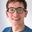## Positive deposit interest calculated in tiers of period AND balance

What

Mambu currently supports tiers defined by either a time period or balance, but in many cases a combination of both period and balance is required.

Why

Some banks offer introductory or ‘teaser’ rates to entice new customers, but which are not intended to be used on balances over a certain figure, or after a certain period of time.

Many deposit taking institutions offer a promotional/introductory rate to attract new clients, e.g. 3% per year for the first 2 months after activation. These rates are also often limited by balance (e.g. only up to \$50000) to avoid clients making large deposits for short term benefit only.

Example:

3% per year for the first 2 months after activation on balances up until \$50,000; for any amounts larger than \$50,000, the standard rate of 1.5% per year applies, even during the initial 2 months.

- 10.10.18: Deposit of \$30,000 made, account activated and introductory rate begins

- 01.11.18: Interest applied, calculated as \$30,000*(3%/365)*22 = \$54.24. New balance = \$30,054.24

- 12.10.18: Deposit of \$25,000 made, new total balance \$30,000 + \$25,000 + 54.24 = \$55,054.25

- 01.12.18: Interest applied, calculated as follows:

(30054.24*(3%/365)*11) + (50000*(3%/365)*19) + (5054.25*(1.5/365)*19) = 27.17 + 78.08 + 3.95 = \$109.20

New balance = 55054.25 + 109.20 = 55163.45

- No deposits/withdrawals in December

- 10.12.18: Introductory rate ends

01.01.19: Interest applied:

(50000*(3%/365)*9) + (5163.45*(1.5%/365)*9) + (55163.45*(1.5%/365)*22) = 36.99 + 1.91 + 49.87 = \$88.77

New balance = 55163.45 + 88.77 = \$55,252.22

•James Anderson
• Nov 8 2018